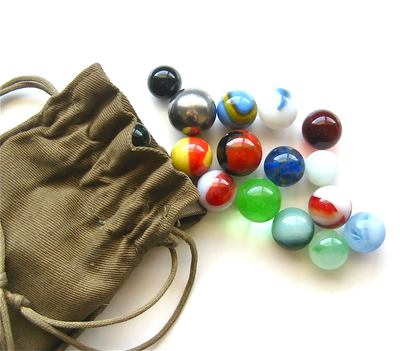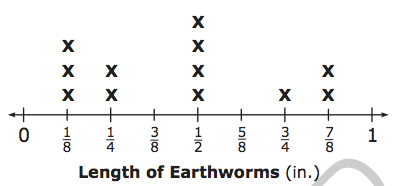MD.3/MD.4
starstarstarstarstarstarstarstarstarstar
by James Wilborn
| 8 Questions1
1
Find the perimeter and area of each figure. Each figure is in inches (in).
2
1
Faye bought some wrapping paper for Christmas that was 8 feet long and 8 feet wide. What is the perimeter of the wrapping paper she bought?
3
1
A piece of plywood was cut so its length was 8 feet by 4 feet. What is the area of the wood?
4
1
An envelope from the post office is 3 inches wide with a total area of 30 square inches. What is the height of the envelope?
5
1
An envelope from the post office is 6 inches wide and 8 inches long. What is the perimeter of the envelope?6
1
If everyone put their M&M's together, how many cups would there be?7
1
Daniel has a set of red, green, and blue marbles. • The red marbles make up exactly ½ of the set. • The set has 2 blue marbles. • The number of green marbles is twice the number of blue marbles. How many marbles are in Daniel’s set?8
1
Using the line plot, what is the difference in the length between the longest earthworm and shortest earthworm?